Courses

# The Multistory Frames with Sidesway - 2 Civil Engineering (CE) Notes | EduRev

## Civil Engineering (CE) : The Multistory Frames with Sidesway - 2 Civil Engineering (CE) Notes | EduRev

The document The Multistory Frames with Sidesway - 2 Civil Engineering (CE) Notes | EduRev is a part of the Civil Engineering (CE) Course Structural Analysis.
All you need of Civil Engineering (CE) at this link: Civil Engineering (CE)

Moment-distribution method

The two-story frame shown in Fig. 22.8a has two independent sidesways or member rotations. Invoking the method of superposition, the structure shown in Fig. 22.8a is expressed as the sum of three systems;

1) The system shown in Fig. 22.8b, where in the sidesway is completely prevented by introducing two supports at E and D. All external loads are applied on this frame.

2) System shown in Fig. 22.8c, wherein the support E is locked against sidesway and joint C and D are allowed to displace horizontally. Apply arbitrary sidesway Δ'1 and calculate fixed end moments in column BC and DE . Using moment-distribution method, calculate beam end moments.

3) Structure shown in Fig. 22.8d, the support D is locked against sidesway and joints B and E are allowed to displace horizontally by removing the support at E . Calculate fixed end moments in column AB and EF for an arbitrary sideswayΔ'2 as  shown the in figure. Since joint displacement as known beforehand, one could use the moment-distribution method to analyse the frame.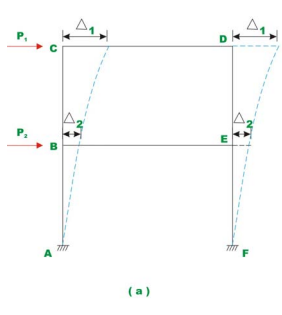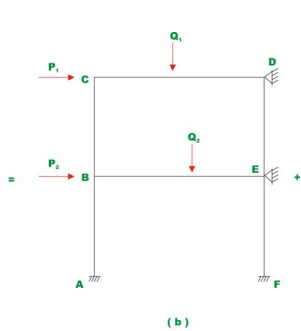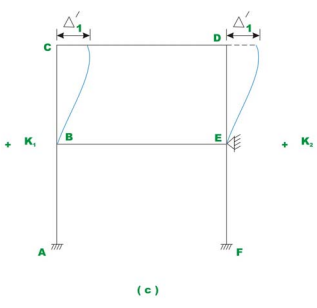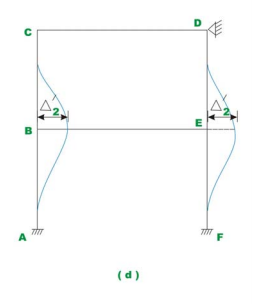Fig.22.8 Two - story frame

All three systems are analysed separately and superposed to obtain the final answer. Since structures 22.8c and 22.8d are analysed for arbitrary sidesway Δ'1 and Δ'2 respectively, the end moments and the displacements of these two analyses are to be multiplied by constants k1 and k2 before superposing with the results obtained in Fig. 22.8b. The constants k1 and k2 must be such that

k1 Δ'1  = Δ1  and k2 Δ'2 = Δ2.                        (22.4)

The constants k1 and k2 are evaluated by solving shear equations. From Fig. 22.9, it is clear that the horizontal forces developed at the beam level CD in Fig. 22.9c and 22.9d must be equal and opposite to the restraining force applied at the restraining support at D in Fig. 22.9b. Thus,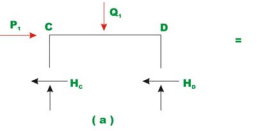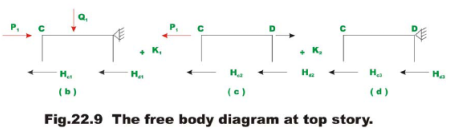k(HC2 + HD2) + k2 (HC3 + HD3) = P1                       (23.5)

From similar reasoning, from Fig. 22.10, one could write,

k1 (HA2 + HF2) + k2 (HA3 + HF3) = P2                              (23.6)

Solving the above two equations, k1 and k2 are calculated.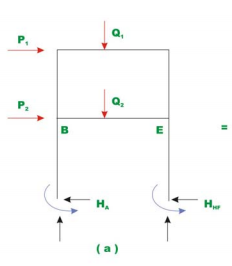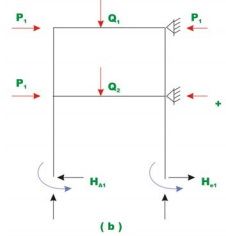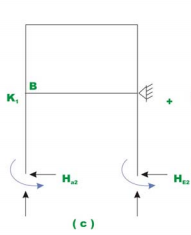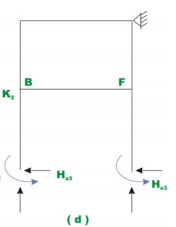Fig.22.10 Free body diagram at the base of Frame.

Example 22.2
Analyse the rigid frame of example 22.1 by the moment-distribution method.

Solution:
First calculate stiffness and distribution factors for all the six members.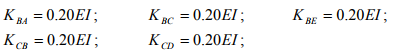(1)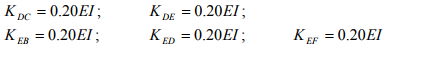Joint B :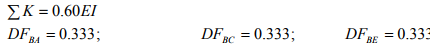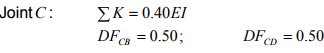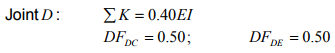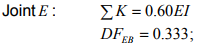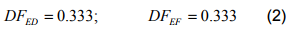The frame has two independent sidesways: Δ1 to the right of CD and to the right of Δ2 BE . The given problem may be broken in to three systems as shown in Fig.22.11a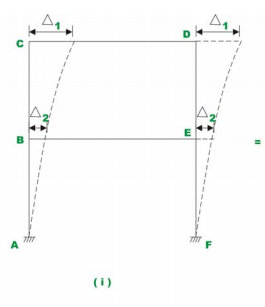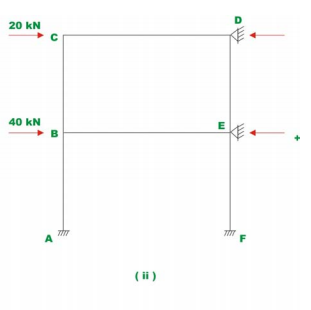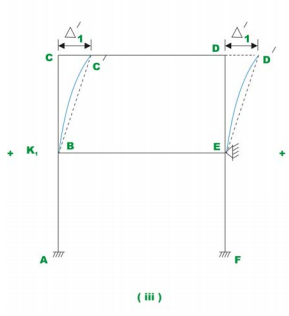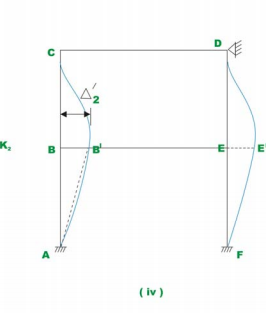Fig. 22.11a Example 22.2

In the first case, when the sidesway is prevented [Fig. 22.10a (ii)], the only internal forces induced in the structure being 20 kN and 40 kN axial forces in member CD and BE and respectively. No bending moment is induced in the structure. Thus we need to analyse only (iii) and (iv).

Case I :

Moment-distribution for sidesway Δ'1 at beam CD [Fig. 22.1qa (iii)]. Let the arbitrary sidesway be Δ'1 = 25/EI . Thus the fixed end moment in column CB and DE due to this arbitrary sidesway is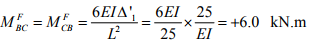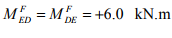(3)

Now moment-distribution is carried out to obtain the balanced end moments. The whole procedure is shown in Fig. 22.11b. Successively joint ,, BCD and E are released and balanced.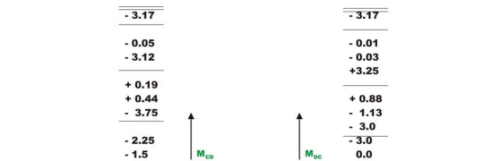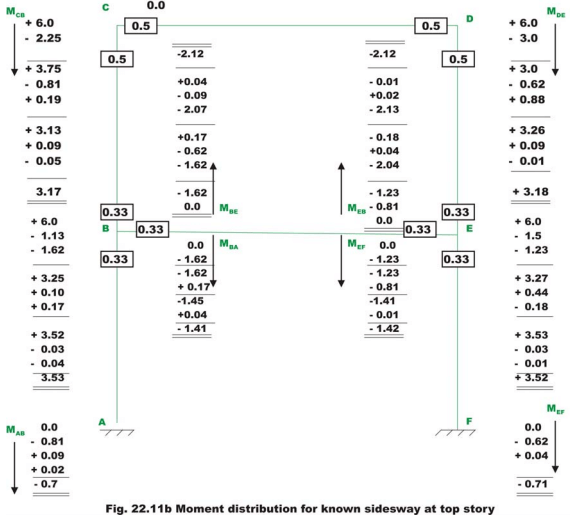From the free body diagram of the column shown in Fig. 22.11c, the horizontal forces are calculated. Thus,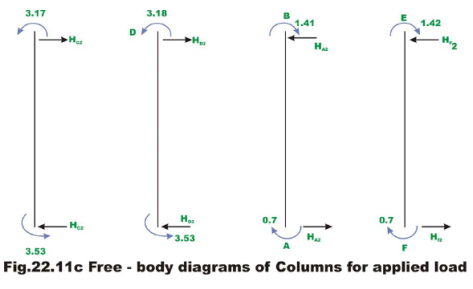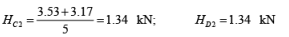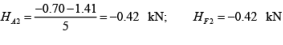(4)

Case II :

Moment-distribution for sidesway Δ'2 at beam BE [Fig. 22.11a (iv)]. Let the arbitrary sidesway be Δ'2 = 25/EI

Thus the fixed end moment in column AB and EF due to this arbitrary sidesway is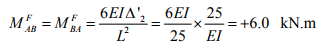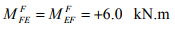(5)

Moment-distribution is carried out to obtain the balanced end moments as shown in Fig. 22.11d. The whole procedure is shown in Fig. 22.10b. Successively joint D, C, B and E are released and balanced.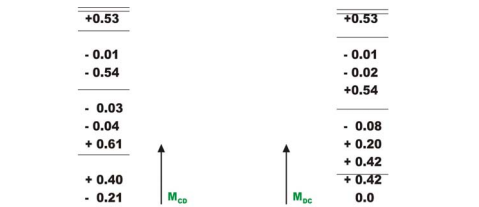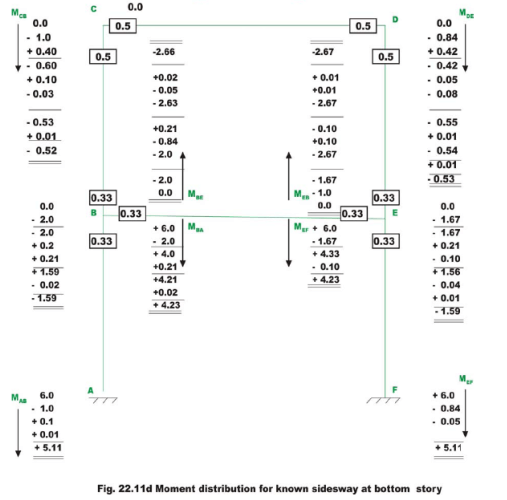From the free body diagram of the column shown in Fig. 22.11e, the horizontal forces are calculated. Thus,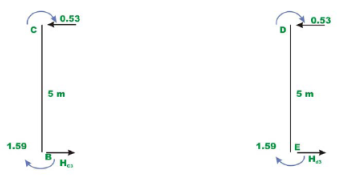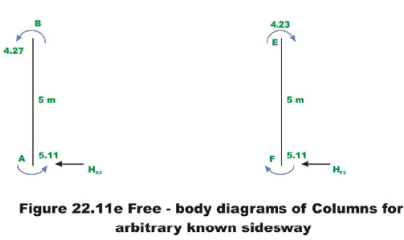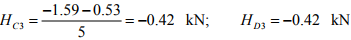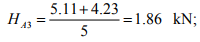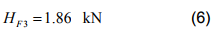For evaluating constants k1 and k2, we could write, (see Fig. 22.11a, 22.11c and 22.11d).

k1 (HC2 + HD2) + k2 (HC3 + HD3) = 20

k1 (HA2 + HF2)+ k2 (HA3 + HF3) = 60

k1 (1.34 +1.34) + k2 (- 0.42 - 0.42) = 20

k1 (- 0.42 - 0.42) + k2 (1.86 +1.86) = 60

k1 (l.34) + k2 (- 0.42) = 10

k1 (- 0.42) + k2 (l.86) = 30

Solving which, k1 = 13.47 k2 = 19.17                             (7)

Thus the final moments are,

MAB = 88.52 kN.m ; MBA = 62.09 kN.m

MBC = 17.06 kN.m; MCB = 32.54. kN.m

MBE = -79.54 kN.m ;MEB = -79.54 kN.m

MCD =-32.54 kN.m ;MDC =-32.54 kN.m

MDE = 32.54 kN.m ; MED = 17.06 kN.m

MEF = 62.09 kN.m ; MFE = 88.52 kN.m                           (8)

Summary

A procedure to identify the number of independent rotational degrees of freedom of a rigid frame is given. The slope-deflection method and the momentdistribution method are extended in this lesson to solve rigid multistory frames having more than one independent rotational degrees of freedom. A multistory frames having side sway is analysed by the slope-deflection method and the moment-distribution method. Appropriate number of equilibrium equations is written to evaluate all unknowns. Numerical examples are explained with the help of free-body diagrams.

Offer running on EduRev: Apply code STAYHOME200 to get INR 200 off on our premium plan EduRev Infinity!

## Structural Analysis

30 videos|122 docs|28 tests

,

,

,

,

,

,

,

,

,

,

,

,

,

,

,

,

,

,

,

,

,

;